# Geometry Problems

Here are a bunch of geometry problems that you can attack with a computer geometry program like Geometer. Most of them are moderately difficult, but you may be able to use some of Geometer's features to help you solve them. Here is a set of Geometer Techniques that may help you.

In fact, I am not 100% positive that all of the problems below are true. I have 99% confidence, but there are a couple of them that I can't solve.

All of the problems here have an associated Geometer diagram that you can download to make it easy to play with the problems. These files will be useless to you unless you have installed Geometer.

Without further ado, here are some problems, described in English. Download the Geometer file to view the diagram.

1.Triangle Center I. Given ΔABC, form its superior triangle by constructing lines parallel to the sides through the vertices opposite those sides. This will produce three more triangles congruent to the original. Construct the circumcircles of the three new triangles and connect the center of each to the opposite vertex with three straight lines. Show that those three lines always meet at a point.

2.Triangle Center II. Given ΔABC, form its superior triangle by constructing lines parallel to the sides through the vertices opposite those sides. This will produce three more triangles congruent to the original. Construct the nine-point circles of the three new triangles and connect the center of each to the opposite vertex with three straight lines. Show that those three lines always meet at a point. (The nine-point circle of a triangle is the circle passing through the midpoints of all three sides.)

3.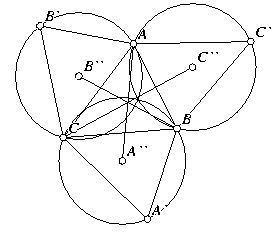Triangle Center III. Given ΔABC, reflect each point across the opposite side and connect that point to the two vertices of the opposite side to form three new triangles. Construct the circumcircles of the three new triangles and connect the center of each to the opposite vertex with three straight lines. Show that those three lines always meet at a point. Also show that the three circumcircles meet at a point.

4.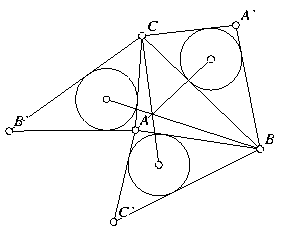Triangle Center IV. Given ΔABC, reflect each point across the opposite side and connect that point to the two vertices of the opposite side to form three new triangles. Construct the incircles of the three new triangles and connect the center of each to the opposite vertex with three straight lines. Show that those three lines always meet at a point.

5.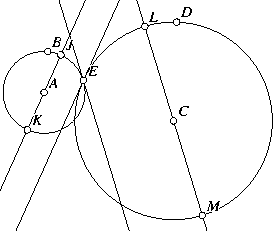Quantum Problem. This problem is from Quantum magazine. Given two circles that intersect in two points, choose one of the points of intersection and construct lines tangent to both circles at that point. Draw diameters of the two circles parallel to the tangent line of the other circle. Show that the four points that are the endpoints of the diameters all lie on a circle.

6.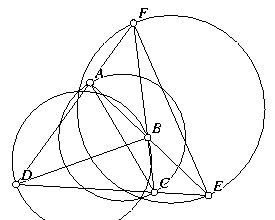Coaxial Circles. If circles are drawn whose diameters are the diagonals of a complete quadrilateral, those circles are coaxial. A complete quadrilateral consists of four general lines and their six intersections. The diagonals are the segments connecting the pairs of intersections that are not already connected. Circles are coaxial if there exists a line (the axis) such that the tangents to the circles from any point on that line where it makes sense to draw tangents are of equal length.

If the circles intersect, the axis passes through the two points of intersection, so if any two of the three coaxial circles intersect, all three of them do in the same two points.

7.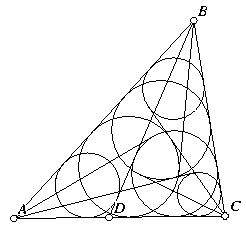Six Circles. This is a problem from the American Mathematical Monthly. Given any ΔABC, select a point D on AC, between A and C. Inscribe a circle in ΔDBC. Now draw the line through A and tangent to that incircle meeting line BC at a point E. Next, inscribe a circle in ΔAEB. Draw the line tangent to that incircle from C, and continue in this way, inscribing circles in the newly-formed triangles and drawing tangents. Show that after six such circles are inscribed, they begin to cycle; the seventh circle is the same as the first.

8.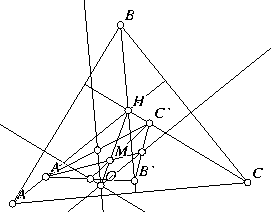Concurrent Perpendiculars. In any ΔABC, let A', B', and C' be points selected arbitrarily on the altitudes dropped from points A, B, and C, respectively. Find the midpoints of ΔA'B'C' and from those three points, drop perpendiculars to the sides of ΔABC. (Drop the perpendicular from the midpoint of A'B' to side AB, et cetera.) Show that those three perpendiculars are concurrent.

9.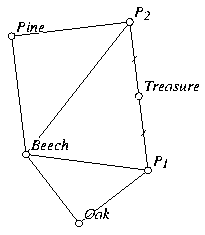Pirate Treasure. Pirates bury treasure with the following instructions:

Start at the beech tree, walk to the oak, turn 90 degrees left and walk the same distance to a point P. Next, start at the beech tree, walk to the pine tree, turn 90 degrees right, and walk the same distance to a point Q. The treasure is at the midpoint of P and Q. But when you get there, the beech tree is missing, but the pine and oak are still standing. How can you find the treasure?

10.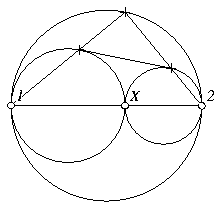Circle Tangent Problem. Two circles are tangent and a third circle surrounds them having the same diameter as their common diameter. A common external tangent to the smaller circles is drawn and it touches the smaller circles in two points. Lines from the endpoints of the diameter of the larger circle are drawn through those two points. Show that those lines meet at a point on the larger circle, and that if a perpendicular is dropped from this point of intersection to the common diameter, it will meet the diameter where the two smaller circles meet.